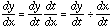Date: Fri, 6 Aug 1999 03:34:24 -0600 (CST) Subject: parametric equation Name: Nicholas Who is asking: Student Question: Show that an equation of the normal to the curve with parametric equations x=ct y=c/t t not equal to 0, at the point (cp, c/p) is : y-c/p=xp^2-cp^3 Hi Nicholas, The slope of the normal to a curve at a point P is -1 divided by the slope of the tangent to the curve at P, and the slope of the tangent to the curve at P is given by the derivative dy/dx at P. Your curve is given in terms of a parameter t and thus you can calculate dy/dx by:Using x=ct and y=c/t find dy/dt and dx/dt and then use the expression above to find dy/dx at the point P(cp,c/p). Use the result to calculate the slope of the normal at P and then write the equation of the line with this slope that passes through P. Cheers Harley

Go to Math Central

To return to the previous page use your browser's back button.